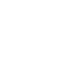Using machine learning and weather data to predict wind turbine power
We obtained 70,000 records of wind-speed data taken from 13 different locations, every 15 minutes for 2 years
In 2019, a Chinese alternative energy company turned to Singuilarex to develop an algorithm to predict wind power generation that would be more accurate than the one they were using. They were looking for a solution that would help them plan the load on the power grid in their region.
We obtained 70,000 records of wind-speed data taken from 13 different locations, every 15 minutes for 2 years.

We also got other weather data, such as:

• Wind direction and speed
• Temperature
• Air humidity
• Atmosphere pressure

The task was to analyze all the data, taking into account the power of the wind turbines. We were looking for the most accurate method to help predict how much energy the turbines produce according to given weather indicators.
Techniques tested in the research
Linear regression, third-order polynomial regression, support vector machine, decision trees, random forest, neural networks.
Choosing the most accurate algorithm of power prediction
We chose the random forest method, as it seemed to be the most accurate.

The criteria for model selection was RMSE (Root Mean Square Error), which measures how much error exists between two data sets.

RMSE was calculated according to the following formula:Where n = the number of cases; Q = actual power; Q' = predicted power; C = max installed capacity.
The model creation process
Our work process on this project was divided into two stages:

1. Using the data for training the retrospective model to predict the wind turbine's power
2. Using the created model for real-time prediction purposes

After the algorithm was created, we deployed the API on the server so that we could drop the weather data and receive, in response, predictions for 4 hours and 72 hours ahead in real-time mode.

Using the created model, we made the first prediction for 4 hours. We then compared the results with the actual data after we received them 4 hours later.

You can see the results of our prediction in the graph:Actual power vs Predicted power over time
After that, we tested our method in real time for the 72-hour interval. The results are shown in the next graph:Actual power vs predicted power over time
The result was a new working algorithm for further predictions.
The results
Our work took 2 weeks and was conducted by three data scientists supervised by a Doctor of Science from Singularex. The challenging part of the project was understanding the Chinese language and ensuring the consistency of the sensor data. We managed to improve the accuracy of the power prediction algorithm from 0,86 to 0,93. Our model was successfully implemented and is still working for planning the load on the region's alternative energy grid.
Get a free consultation from our expert
MORE CASE STUDIES